# Jarosite

Steven Dutch, Professor Emeritus, Natural and Applied Sciences, University of Wisconsin - Green Bay

Jarosite is a reasonably common but not especially significant mineral on Earth, where it forms by the weathering of iron sulfides. However, it has been found to exist on Mars, where it indicates that Mars once had surface water. The formula of jarosite is KFe3+3(OH)6(SO4)2

Jarosite has a nice geometric structure consisting of fairly regular coordination polyhedra. Especially interesting are the icosahedrally-coordinated potassium atoms, linked by iron octahedra and sulfate tetrahedra. In these figures, potassium is purple, iron is orange and sulfur (of course) is yellow. Hydrogen atoms are red and O-H bonds are green

Probably the neatest thing about jarosite is the icosahedral coordination of the potassium. Despite their high symmetry, icosahedra are relatively uncommon as coordination polyhedra because five-fold symmetry is not crystallographically possible. Thus, coordination icosahedra exploit some of the lower symmetries of icosahedra. In the case of jarosite, that's a threefold symmetry axis.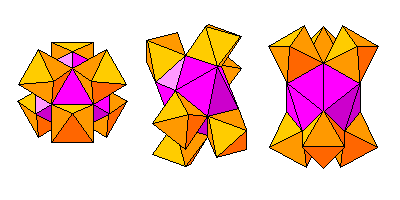At left are three views of ferric iron octahedra joined to the potassium icosahedron. Three icosahedra enclose a polar triangular face, and the remaining triangles form a zigzag equatorial band. Although Pauling's Rules frown on face-to- face polyhedra, the low charge (+1) and large ionic radius of potassium make it possible here.The sulfur tetrahedra share vertices around the equator.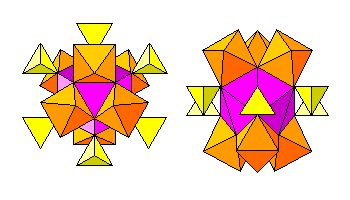Here are views showing the coordination of the iron and sulfur atoms.Above are the potassium octahedra, looking down the three-fold (c-) axis. Layers of octahedra are offset with a three-layer repeat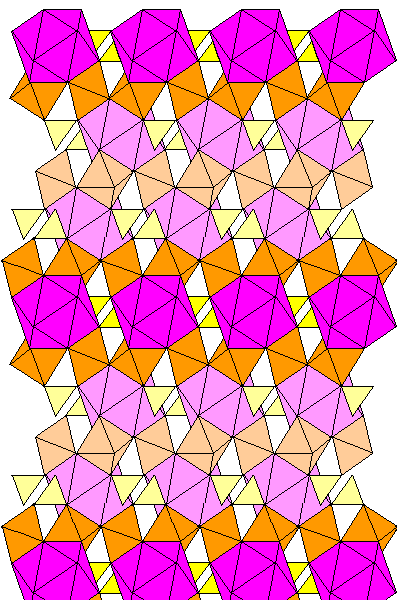The view at left looks along the a-axis perpendicular to the c-axis. Successive layers of potassium icosahedra are linked by iron octahedra. Within planes, potassium atoms are linked with sulfur tetrahedra. Successive layers are offset. Lighter colors denote atoms behind the plane of the diagram.Above is a layer of potassium, showing the linkages of the sulfur and ferric iron atoms. Only the ferric iron atoms below the plane of the diagram are shown, except for the potassium atom at extreme lower left.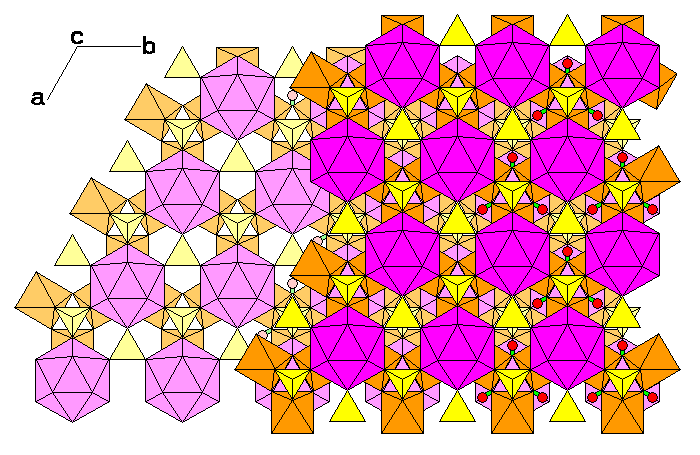This diagram shows two layers of the jarosite structure, again showing only ferric iron atoms below the potassium layers.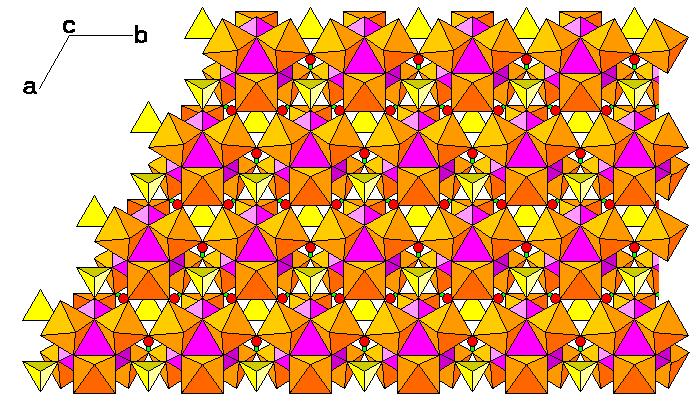The diagram above shows a potassium layer with ferric iron atoms above and below the potassium layer.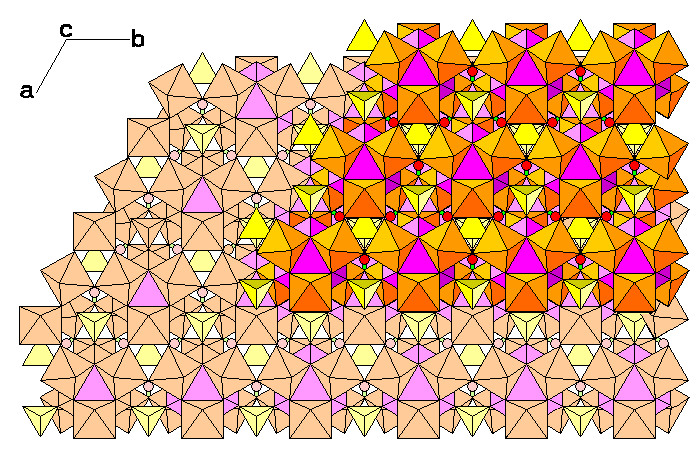The diagram above shows two complete layers of the jarosite structure.This diagram is a view normal to the a-c plane and shows how rows of potassium atoms step back from layer to layer.

Created 15 October 2009, Last Update